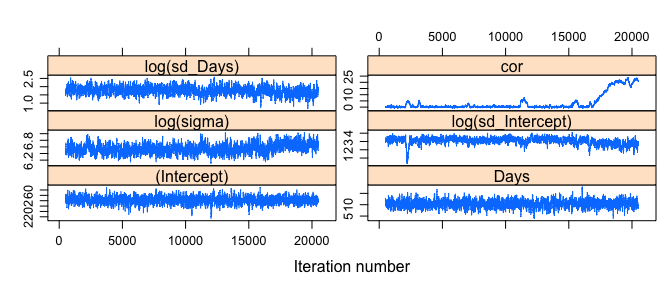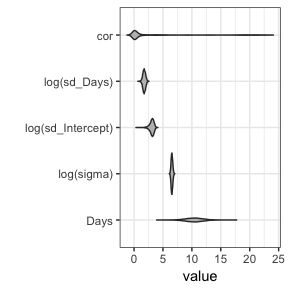# post-hoc MCMC with glmmTMB

#### 2018-05-10

library(glmmTMB)
library(MCMCpack)
library(coda)
library(lattice)
library(ggplot2); theme_set(theme_bw())
library(reshape2)

Fit basic model:

data("sleepstudy",package="lme4")
fm1 <- glmmTMB(Reaction ~ Days + (Days|Subject),
sleepstudy)

Set up for MCMC: extract coefficients and variance-covariance matrices as starting points.

## FIXME: better way for user to extract full coefs?
rawcoef <- with(fm1$obj$env,last.par[-random])
stopifnot(all.equal(c(fm1$obj$fn(rawcoef)),
-c(logLik(fm1)),
tolerance=1e-7))
## log-likelihood function
## (MCMCmetrop1R wants *positive* log-lik)
fn <- function(x) -fm1$obj$fn(x)
V <- vcov(fm1,full=TRUE)
s1 <- system.time(m1 <- MCMCmetrop1R(fn,rawcoef,V=V))

(full run takes 89 seconds)

Post-process for convenience:

colnames(m1) <- c(names(fixef(fm1)[]),
"log(sigma)",
c("log(sd_Intercept)","log(sd_Days)","cor"))
xyplot(m1,layout=c(2,3))The trace plot for the correlation is extremely problematic; the effective sample size backs this up, as would any other diagnostics we did.

print(effectiveSize(m1),digits=3)
##       (Intercept)              Days        log(sigma) log(sd_Intercept)
##            1155.9            1019.4             371.8             139.0
##      log(sd_Days)               cor
##             507.9               4.3

Leaving out the intercept because it’s on a very different scale (an alternative would be to facet to give each variable its own scale, but if we want violin plots + faceting + horizontal layout we would need to pull in the ggstance package …)We can do a little better, e.g. by setting the tune argument of MCMCmetrop1R to tune=c(1,1,1,1,1,100), but the parameter may simply be very poorly determined.

## To do

• diagnose and solve mixing for cor parameter
• better description/tools for end users to get at raw parameters and understand their meanings (especially variance-covariance parameters)
• more complex example - e.g. Owls
• multi-chain/Gelman-Rubin example? (Don’t think MCMCmetrop1R can do this; could easily cook up a simple multivariate M-H sampler, but wouldn’t want to reimplement all the bells and whistles, e.g. adaptive tuning …)